Passionate about Data Science, AI, Programming & Math | Owner of ∇² https://www.nablasquared.com/

# How to use C code in Python

## … and should we do this?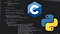Background image by Johnson Martin from Pixabay
1. Reusing existing C code from a Python program
2. Speed up your Python program
3. Do some low-level stuff that cannot be done directly in Python

# How to prettify your code in a Jupyter Notebook

## Use the Code Prettify Jupyter Extension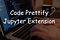Background image by Free-Photos from Pixabay

## Installation

`pip install jupyter_contrib_nbextensions`

# What are GANs?

## A brief introduction to Generative Adversarial Networks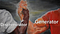Image source: https://meme-creator.com/meme/54/epic-handshake

# How to change the autosave interval in Jupyter Notebooks

## You can use this Jupyter extension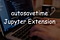Background image by Free-Photos from Pixabay

# How to Implement Logistic Regression with PyTorch

## Understand Logistic Regression and sharpen your PyTorch skills

• We will rewrite the logistic regression equation so that we turn it into a least-squares linear regression problem with different labels and then, we use…

# How to Implement Logistic Regression with TensorFlow

## TL; DR

`model = tf.keras.models.Sequential([    tf.keras.layers.Dense(1, activation='sigmoid')])model.compile(loss='bce')model.fit(x_train, y_train, epochs=100)`

# Are you tired of scrolling down through logs when training an ML model?

## Try this Jupyter extension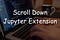Background image by Free-Photos from Pixabay

# The best 2 books to learn C & C++

## How I learned more in 3 months rather than 4 years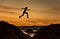Image by Sasin Tipchai from Pixabay

# How to code Logistic Regression from scratch with NumPy

## Sharpen your NumPy skills while learning Logistic Regression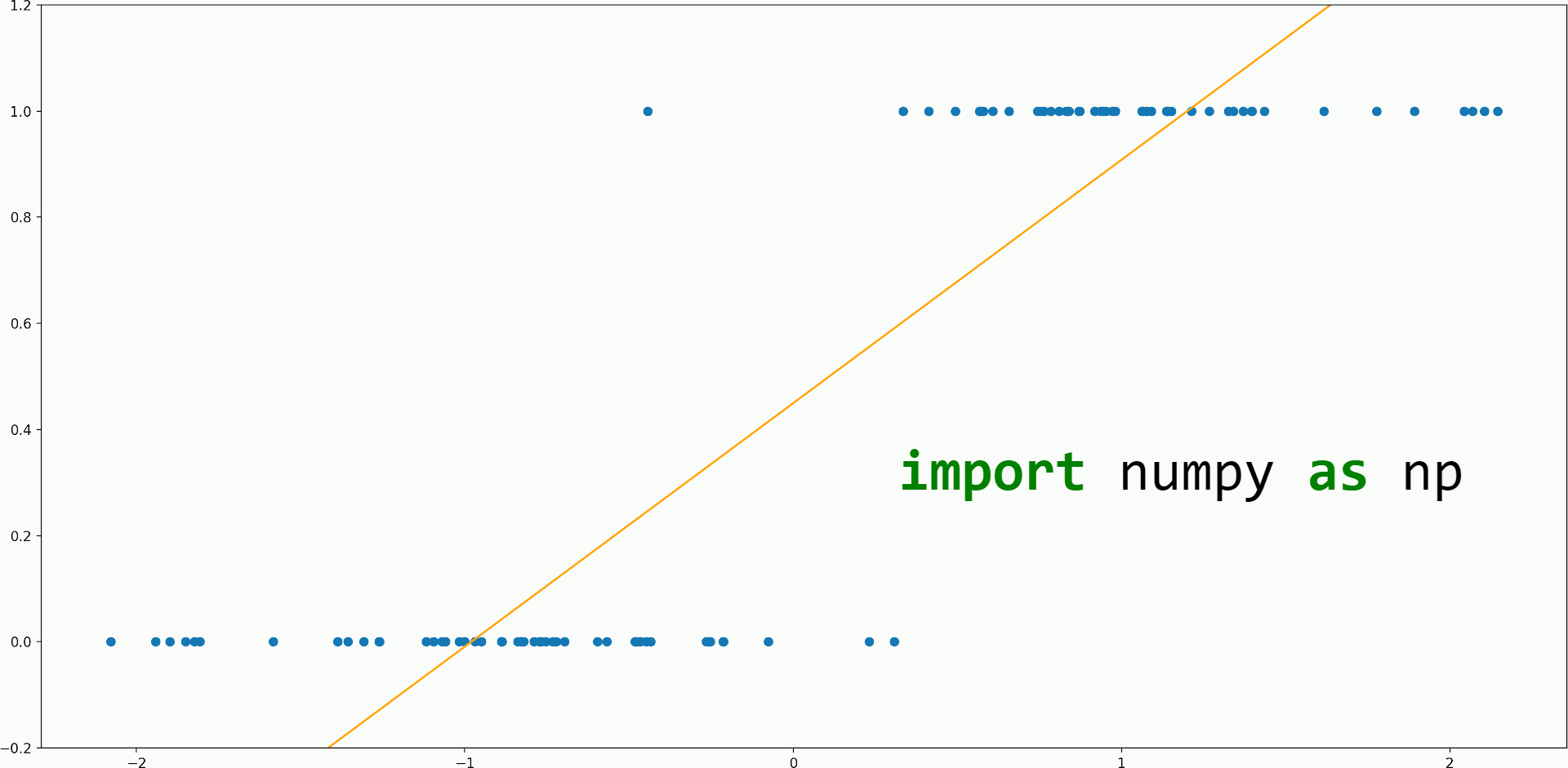Image by author
• We will rewrite the logistic regression equation so that we turn it into a least-squares linear regression problem with different labels and then, we use the closed-form formula to find the weights:

# Understanding Logistic Regression

## The math of this method explained in detail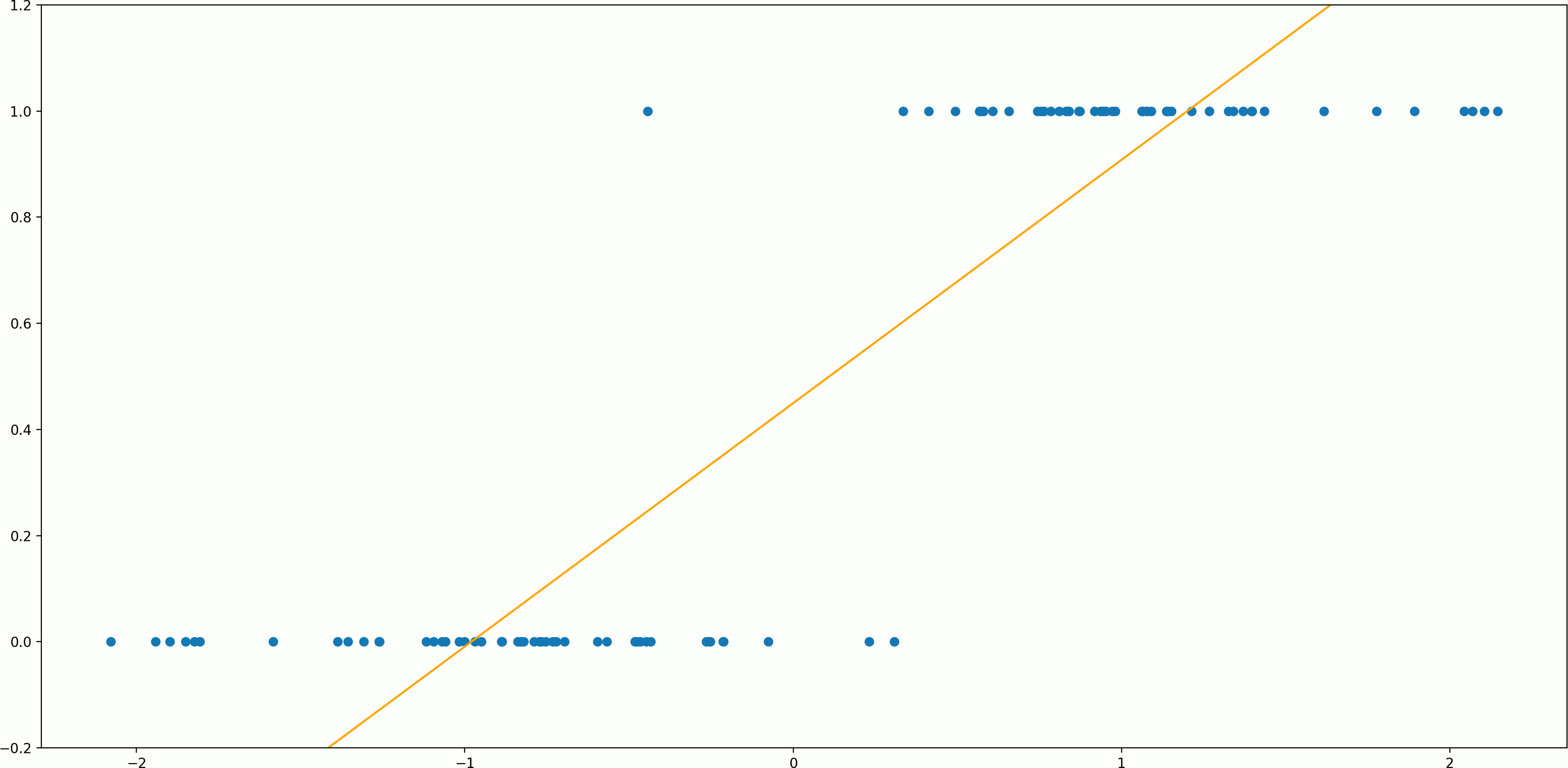Image by author